# References and Data Structures

A reference is an indirect link to a value, an atom or an array. A variable can contain a single reference to a value, or it can contain an array of references. Variables and arrays can themselves contain references, nested to any depth. This lets you create complex data structures, such as linked lists, trees, and non-rectangular structures. Use of references is provided by two operators:

• \e is the reference operation. It creates a reference to the value of expression «e».
• #e is the dereference operation. It obtains the value referred to by «e». If «e» is not a reference, it issues a warning and returns Null.

An example:

Variable M
Definition: 100
Variable Ref_to_M
Definition: \M

The result of Ref_to_M looks like this: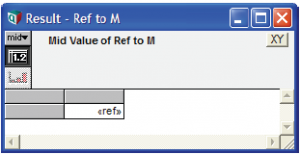You can double-click the cell containing «ref» to view the value referenced, in this case: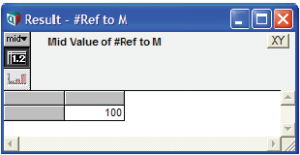You can also create an array of references. Suppose:

Index K
Definition: 1..5
Variable Ksquare
Definition: K^2
Ksquare →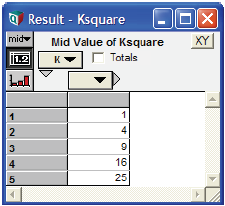Variable Ref_to_Ksquare
Definition: \Ksquare
Ref_to_Ksquare →If you click the «ref» cell, it opens: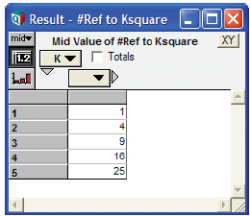You can also create an array of references from an array, for example:

Variable Ref_Ksquare_array
Definition: \[]Ksquare
Ksquare →

The empty square brackets [ ] specify that the values referred to have no indexes, i.e., they are atoms. You can now click any of these cells to see what it refers to.Clicking the third cell, for example, gives: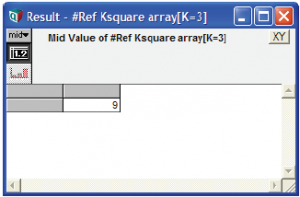## Managing indexes of referenced subarrays: \[i, j,...] e

More generally, you can list in the square brackets any indexes of «e» that you want to be indexes of each subarray referenced by the result. The other indexes of «e» (if any) are used as indexes for the referencing array. Thus, in the example above, since there were no indexes in square brackets, the index K was used as an index of the reference array. If instead we write:

\[K] Ksquare →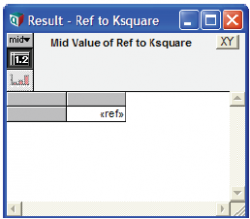It creates a similar result to \Ksquare, since K is the only index of Ksquare.

To summarize:

Construct Meaning
\e Creates a reference to the value of expression «e», whether it is an atom or an array.
\[]e Creates an array indexed by all indexes of «e» containing references to all atoms from «e».
\[i]e Creates an array indexed by any indexes of «e» other than «i» of references to subarrays of «e» each indexed by «i».
\[i, j…]e Creates an array indexed by any indexes of «e» other than «i», «j» … of references to subarrays of «e» each indexed by «i», «j» ….

In general, it is better to include the square brackets after the reference operator, and avoid the unadorned reference operator, as in the first row of the table. Being explicit about which indexes to include generally leads to expressions that array abstract as intended.

## IsReference(x)

IsReference Is a test to see whether its parameter «x» is a reference. It returns True (1) if «x» is a reference, False (0) otherwise.

## Using references for linked lists: Example functions

Linked lists are a common way for programmers to represent an ordered set of items. They are more efficient than arrays when you want often to add or remove items, thereby changing the length of the list (which is more time consuming for arrays). In Analytica, we can represent a linked list as an element with two elements, the item — that is, a reference to the value of the item — and a link — that is, a reference, to the next item:

Definition: ['Item', 'Link']
Function LL_Put(x, LL)
Description: Puts item x onto linked list LL.
Definition: \Array(Linked_List, [\x, LL])
Function LL_Get_Item(LL)
Description: Gets the value of the first item from linked list LL.
Definition: # Subscript(#LL, Linked_list, 'Item')
Function LL_length(LL)
Parameters: (LL: Atom)
Description: Returns the number of items in linked list LL
Definition: Local len := 0; While (IsReference(LL)) BEGIN LL := Subscript(#LL, Linked_List, "Next"); len := len + 1 END; len

Function LL_from_array(a, i)
Parameters: (a; i: Index)
Description: Creates a linked list from the elements of array a over index i
Definition: Local LL := NULL; LocalIndex iRev := IndexLength(i) .. 1; For j := iRev Do LL := LL_Push(LL, Slice(a, i, j)); LL

See Linked List Library.ana in the Libraries folder for these and other functions for working with linked lists.